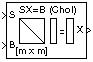# Cholesky Solver

Solve SX=B for X when S is square Hermitian positive definite matrix

## Library

Math Functions / Matrices and Linear Algebra / Linear System Solvers

`dspsolvers`

•## Description

The Cholesky Solver block solves the linear system SX=B by applying Cholesky factorization to input matrix at the `S` port, which must be square (M-by-M) and Hermitian positive definite. Only the diagonal and upper triangle of the matrix are used, and any imaginary component of the diagonal entries is disregarded. The input to the `B` port is the right side M-by-N matrix, B. The M-by-N output matrix X is the unique solution of the equations.

A length-M vector input for right side B is treated as an M-by-1 matrix.

### Response to Nonpositive Definite Input

When the input is not positive definite, the block reacts with the behavior specified by the Non-positive definite input parameter. The following options are available:

• `Ignore` — Proceed with the computation and do not issue an alert. The output is not a valid solution.

• `Warning` — Proceed with the computation and display a warning message in the MATLAB® Command Window. The output is not a valid solution.

• `Error` — Display an error dialog box and terminate the simulation.

### Note

The Non-positive definite input parameter is a diagnostic parameter. Like all diagnostic parameters on the Configuration Parameters dialog box, it is set to `Ignore` in the code generated for this block by Simulink® Coder™ code generation software.

## Algorithm

Cholesky factorization uniquely factors the Hermitian positive definite input matrix S as

`$S=L{L}^{\ast }$`

where L is a lower triangular square matrix with positive diagonal elements.

The equation SX=B then becomes

`$L{L}^{\ast }X=B$`

which is solved for X by making the substitution $Y={L}^{\ast }X$, and solving the following two triangular systems by forward and backward substitution, respectively.

`$LY=B$`
`${L}^{\ast }X=Y$`

## Parameters

Non-positive definite input

Response to nonpositive definite matrix inputs: `Ignore`, `Warning`, or `Error`. See Response to Nonpositive Definite Input.

## Supported Data Types

• Double-precision floating point

• Single-precision floating point

 Autocorrelation LPC DSP System Toolbox Cholesky Factorization DSP System Toolbox Cholesky Inverse DSP System Toolbox LDL Solver DSP System Toolbox LU Solver DSP System Toolbox QR Solver DSP System Toolbox `chol` MATLAB

See Linear System Solvers for related information.

Watch now